# Real numbers and complex numbers

• B
• grzz
I do not see the relevance of the discussion about the existence of numbers to the original post. The main point is that the two methods given in the original post do not give the same answer, and the explanation for this is that the rules for manipulating square roots do not apply to complex numbers in the same way as they do to real numbers.Whether numbers "exist" or not is a philosophical question that is beyond the scope of this forum. In mathematics, we simply define different number systems and study their properties and relationships.

#### grzz

TL;DR Summary
To find √(-2)√(-3).

Method 1.
√(-2)√(-3) = √( (-2)(-3) ) = √(6).

Method 2.
√(-2)√(-3) = √( (-1)(2) )√( (-1)(3) ) = √((-1)√(2)√(-1)√(3) = i√(2)i√(3) = (i)(i)√(2)√(3) = -1√( (2)(3) ) =-√6.

Why don't the two methods give the same answer?
Thanks for any help.

Last edited by a moderator:
grzz said:
Summary:: Why don't the answers agree?

To find √(-2)√(-3).

Method 1.
√(-2)√(-3) = √( (-2)(-3) ) = √(6).

Method 2.
√(-2)√(-3) = √( (-1)(2) )√( (-1)(3) ) = √((-1)√(2)√(-1)√(3) = i√(2)i√(3) = (i)(i)√(2)√(3) = -1√( (2)(3) ) =-√6.

Why don't the two methods give the same answer?
Thanks for any help.
Because the first one is wrong! ##\sqrt{wz} \ne \sqrt w \sqrt z##

Last edited by a moderator:
•DaveE
When you start using complex numbers, the usual simple rules for the square root need to be replaced by more sophisticated methods. You often need to consider multiple values for the square root and determine which are valid in that specific case.

•DaveE
Basically the problem with square roots is that they are not single valued. Using rules doesn't change that fact. The methods you are using go through different branches. The rules might restrict the branches used, but mathematics (as an abstract being) doesn't necessarily obey man-made rules.

•FactChecker

I'll just add that, as a real world practicing EE that uses complex numbers all the time, you are nearly always better off thinking of them in polar coordinates along with the knowledge that the arguments can "wrap around". Yes, sometimes you need to switch to rectangular coordinates (addition, for example), but when you get confused, always check the polar notation, it's usually simpler, IMO.

•WWGD, 2milehi and FactChecker
Well, this wouldn't be a problem, if we would introduce ##\mathbb{C}## as ##\mathbb{R}[x]/(x^2+1).##

\begin{align*}
\sqrt{-2}&=ax+b \;(a\neq 0)\Longrightarrow -2=a^2x^2+2abx+b^2 =-a^2+2abx+b^2 \Longrightarrow b=0\wedge a=\sqrt{2}\\
\sqrt{-3}&=cx+d \;(c\neq 0)\Longrightarrow d=0\wedge c=\sqrt{3}\\
\sqrt{-2}\sqrt{-3}&=\sqrt{2}x \cdot \sqrt{3}x =\sqrt{6}x^2=-\sqrt{6}
\end{align*}

•etotheipi
##-2 =-a^2+2abx+b^2 \Longrightarrow b=0\wedge a=\sqrt{2}##

I don't see how this would follow. ##b=0\wedge a=-\sqrt{2}## is a solution, too. You still get the same ambiguity the complex square root has.

In the long run, it is worth it to get used to multi-valued functions and how to deal with them. They will occur in other situations. This example is about as simple an introduction as you can get.

mfb said:
I don't see how this would follow. ##b=0\wedge a=-\sqrt{2}## is a solution, too. You still get the same ambiguity the complex square root has.
You are right. But this is the ambiguity in the notation of ##\sqrt{a}##, not of complex numbers. Your argument uses that ##\pm \sqrt{a}## both solve ##x^2=a## which assigns four possibilities to ##\sqrt{-2}\sqrt{-3}.## It has nothing to do with complex numbers.

I thank all those who posted their replies.

But am I correct to argue as follows?

Using only real numbers,
√( (-2)(-3) ) = √6 is correct but √(-2)√(-3) does not exist as a real number.

Hence Method 1 and Method 2 in my first post cannot be compared.

•FactChecker
grzz said:
But am I correct to argue as follows?

Using only real numbers,
√( (-2)(-3) ) = √6 is correct but √(-2)√(-3) does not exist as a real number.
Yes, correct. (-2)(-3) = 6, so you are taking the square root of a positive number. In the 2nd example both square roots represent complex (pure imaginary) numbers.
grzz said:
Hence Method 1 and Method 2 in my first post cannot be compared.
Yes.

•FactChecker
Now the math is more clear in my mind.
Thanks Mark44.

"Using only real numbers,
√( (-2)(-3) ) = √6 is correct but √(-2)√(-3) does not exist as a real number."

I guess I see this differently. I agree that both individual square roots are not real, but the question asks about their product, which is real, i.e. sqrt(-2) =± i.sqrt(2) and sqrt(-3) = ±i.sqrt(3), so sqrt(-2).sqrt(-3) = ±i^2.sqrt(2).sqrt(3) = ±sqrt(6), which is a real number.

I.e. the two factors do not exist as real numbers, but their product does. So we are not disagreeing about the math, but about the meaning of the sentence. I.e. to me, the product √(-2)√(-3) does exist as a real number, namely "the" real number ±sqrt(6). (It is not unique, but it is real.)

mathwonk said:
I.e. the two factors do not exist as real numbers, but their product does. So we are not disagreeing about the math, but about the meaning of the sentence. I.e. to me, the product √(-2)√(-3) does exist as a real number, namely "the" real number ±sqrt(6). (It is not unique, but it is real.)
I am uncomfortable talking about the multiplication of numbers that do not exist. I think that a more systematic approach is required to call it proper mathematics.

•pbuk
sorry, i considered my post unhelpful and thought i had deleted it.

now i am tempted to make things worse, and point out that (even "real") numbers do not actually exist.

mathwonk said:
sorry, i considered my post unhelpful and thought i had deleted it.

now i am tempted to make things worse, and point out that (even "real") numbers do not actually exist.
Could you justify what you say? Do natural numbers exist or perhaps correspond sometimes to properties of real things?

Complex numbers can correspond to points of a complex plane, real numbers can correspond to points of a real line if i remember correctly from the books i have read. So, what do you want to say when you say they do not exist? In what way do you mean it?

Natural numbers can be points of a real line but discretely distributed, not continuously like the real numbers as i have concluded.

Does mathematical quantity exist or does mathematical space exist or do
they correspond sometimes to properties of real things? I think mathematical quantity sometimes correspond to properties of real things but mathematical space does not perhaps at all and i think this can be justified and understood easily, at least for myself and perhaps other mathematicians. Euclidean 3 dimensional space though can be visualised but this visualisation is not according to the definitions of its objects.

Other mathematicians obviously have way more knowledge and abilities in problem solving and concluding in mathematics and physics better than i do in my opinion, so they should be able to do this.

Math may be used for for physics, other sciences or technology but it does not mean in my opinion and as i have justified that it necessarily corresponds to properties of real things.It may be connected to properties of real things, but that is different from saying it corresponds to properties of real things.

Last edited by a moderator:
I am compelled to address the question: Do complex numbers exist?

In language, or Math, whether something exists or not depends on what it represents.

In my world of electronics, for example, it is convenient to define a generalized impedance as a complex number. This number represents a magnitude and phase shift between the voltage and current. These are two real quantities that could be represented as a 2-D vector, if you forbade the use of complex arithmetic. But these 2-D vector quantities also satisfy the requirements of a number (identities, inverses, zeros, etc.). So we use them because the arithmetic is easier.

OTOH, if you are counting apples in a basket complex numbers make no sense and don't "exist".

"The teaching is merely a vehicle to describe the truth. Don’t mistake it for the truth itself. A finger pointing at the moon is not the moon. The finger is needed to know where to look for the moon, but if you mistake the finger for the moon itself, you will never know the real moon. The teaching is like a raft that carries you to the other shore. The raft is needed, but the raft is not the other shore. An intelligent person would not carry the raft around on his head after making it across to the other shore." - Thich Nhat Hanh

•PeroK
DaveE said:
I am compelled to address the question: Do complex numbers exist?

Whether something exists or not depends on what it represents.
existence ##\neq## what it represents . This can be justified in my opinion. Especially when talking about knowledge.

trees and plants said:
existence ##\neq## what it represents . This can be justified in my opinion. Especially when talking about knowledge.
So, you may ask "Do you believe in God?" My answer is God exists now because you just referenced it in your question. We may not agree or completely understand what it is, but it exists because we are talking about it.

So we'll agree to disagree. Representation begets existence, at least in some sense.

mathwonk said:
I.e. to me, the product √(-2)√(-3) does exist as a real number, namely "the" real number ±sqrt(6). (It is not unique, but it is real.)
The solution is unique:
\begin{align} x =& \sqrt{-2}\sqrt{-3} \\ =& \sqrt{(-1)2}\sqrt{(-1)3} \\ =& i\sqrt{2}i\sqrt{3} \\ =& i^2\sqrt{(2)(3)} \\ =& -\sqrt6 \end{align}

FactChecker said:
I am uncomfortable talking about the multiplication of numbers that do not exist. I think that a more systematic approach is required to call it proper mathematics.
Yes: 'x does not exist as a real number' is not a mathematical statement (we would say 'x is not an element of the set of real numbers', or just 'x is not a real number').
mathwonk said:
now i am tempted to make things worse, and point out that (even "real") numbers do not actually exist.
DaveE said:
In language, or Math, whether something exists or not depends on what it represents.
None of the things we use in mathematics 'exist' until we create them from axioms: that way we don't have to make assumptions about how they are going to behave.

So we create the integer '2' as the successor of '1', not as the representation of how many things we have if we have two things (discussions elsewhere in PF about equivalence classes notwithstanding).

•PeroK
I also started to say that sqrt(-2) = i.sqrt(2) until I realized that sqrt(-2) means literally anything whose square is 2, so it could also be -i.sqrt(2), but I think I made that clear.

as far as talking about a product being a real number when the factors are not, do you believe that the product of some non - real complex numbers can be a real number? i.e. for example does i^2 exist as a real number? after all it does equal -1. would you say the equation i^2 = -1 makes no sense because the number i does not exist?

as to whether numbers exist, do you believe an infinite set exists? even the integers are an infinite set, but what in the universe is actually infinite? in the class i audited on this topic (constructing, or defining integers, rationals, and eventually real numbers, taught by george mackey) the first unprovable assumption was to assume an infinite set exists. I admit it surprized me at the time that this was considered unprovable.

forgive me for abetting this opinionated discussion, which will no doubt live on and on.

•jim mcnamara
The history of complex numbers started with their use to give the correct answers even though they were not understood as an actual number system. They could be used as a "trick" that disappeared from the final result which was a valid real-number solution. I would hesitate to go back in history to that practice and still call it "mathematics".
That is what I feel is happening if we say that ##\sqrt {-2} \sqrt {-3} = \pm \sqrt 6## without a proper understanding of the individual factors on the left.

Last edited:
mathwonk said:
I also started to say that sqrt(-2) = i.sqrt(2) until I realized that sqrt(-2) means literally anything whose square is 2
I'm pretty sure you meant "anything whose square is -2" ...

Mark44 said:
I'm pretty sure you meant "anything whose square is -2" ...
But that would still be wrong. Anything whose square is -2 is a square root of -2, but the expression ## \sqrt{-2} ## refers to the principal square root of -2 which is unique. I'm sure we all know this but it seems to have got lost somewhere in this thread.

https://mathworld.wolfram.com/SquareRoot.html

•hutchphd and PeroK
pbuk said:
None of the things we use in mathematics 'exist' until we create them from axioms: that way we don't have to make assumptions about how they are going to behave.
"Existence is not a predicate"

One can ask whether a set is non-empty. One cannot ask whether a particular entity exists. In proper mathematical discourse, before you can give a label to an entity, you have to have some assurance that there is such an entity.

For instance, before one can say "Let x be an element of the set of natural numbers" one must have an assurance that the set of natural numbers is non-empty

jbriggs444 said:
"Existence is not a predicate"

One can ask whether a set is non-empty. One cannot ask whether a particular entity exists. In proper mathematical discourse, before you can give a label to an entity, you have to have some assurance that there is such an entity.

For instance, before one can say "Let x be an element of the set of natural numbers" one must have an assurance that the set of natural numbers is non-empty
"Let ##p## be an even prime with ##p > 2##" is perfectly valid and could well be the basis of a proof by contradiction.

PeroK said:
"Let ##p## be an even prime with ##p > 2##" is perfectly valid and could well be the basis of a proof by contradiction.
A proof by contradiction is indeed a special case. One gets to assert a counter-factual (such as that the set of even primes > 2 is non-empty) which justifies the instantiation of an element of the set. The contradiction that is then obtained justifies a conclusion is that the set of even primes > 2 is empty.

If one proceeds in this manner, there was an assurance that p existed when we instantiated it.

Last edited:
IMO, the discussion of the existence of a particular number system is misguided. Whether we can construct an irrational measurement like ##\pi## in a finite number of steps is not relevant to whether it exists. If there is a circle of radius 1, then the circumference exists. Its existence does not depend on our physical ability to measure it or on our understanding of it. In general, our acceptance of the existence of a new number system usually occurs only after it has forced itself on us in some way.

Last edited:
•jbriggs444
@pbuk. Excellent of you to clarify what you mean here by the notation. That is the only way to communicate effectively. As to whether "all of us" share this convention, I myself have been a professional mathematician all my life (over 40 years) and cannot recall ever hearing of this notation being restricted to the "principal square root of -2", by which I presume you mean the one encountered first on the circle of that radius, when starting on the positive real line and traveling counterclockwise. In general a common source of confusion is to assume everyone uses the same definitions without giving the one you use.

I now realize I myself made the same error I refer to when I wrote sqrt(2) and tacitly assumed it was the positive square root only! If my own definition were what I said it was, then sqrt(-2) = i.sqrt(2) would actually contain both possibilities! (Of course one would need to clarify the meaning of "equals", to include sets of two elements.)

mathwonk said:
@pbuk. As to whether "all of us" share this convention, I myself have been a professional mathematician all my life (over 40 years) and cannot recall ever hearing of this notation being restricted to the "principal square root of -2"

I can't remember whether it was as universally agreed on 40 years ago, but in 2021 I think you will find this is the common definition:

"Any nonnegative real numberhas a unique nonnegative square root; this is called the principal square root and is written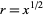or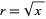" etc. https://mathworld.wolfram.com/SquareRoot.html

"## \sqrt 2 ##: Positive number whose square is ## 2##". https://mathvault.ca/hub/higher-math/math-symbols/

"one defines ## \sqrt 2 ## ... by proving that there is exactly one positive real number that squares to 2. Then ## \sqrt 2 ## is defined to be this number." Princeton Companion to Mathematics

etc.

•mathwonk
As usual, I like the version on nLab:
https://ncatlab.org/nlab/show/square+root
If ##K## is a monoid (which we write multiplicatively) and ##x## is an element of ##K##, then the element ##x^2## is the square of ##x##. Conversely, if ##x^2=y##, then ##x## is a square root of ##y##.

If ##K## is an integral domain, then (in classical mathematics) ##x## and ##−x## are the only square roots of ##x^2##. If ##y## has a square root, then we often denote its square roots together as ##\pm\sqrt{y}##, although there is no meaning of ##\sqrt{y}## itself.

If ##K## is a linearly ordered field, then every element ##y## has a unique nonnegative square root if it has a square root at all; this is the principal square root of ##y## and denoted ##\sqrt{y}##.

•pbuk, mathwonk and jbriggs444
I like the idea of a principal square root of a positive real (that's the one I was assuming in my post for sqrt(2), and I have heard about that) better than the idea of a "principal" complex square root of a negative number. In fact the more I think about it, it seems to me there is really no way to define a principal square root of a negative number without referring to a specific model of the complex numbers, one in which a preferred square root of -1 has been chosen and given a specific name. I.e. in the abstract complex numbers, defined merely as an algebraic closure of the reals, there is no preferred square root of -1, hence no distinguished element to call i.

When I defined the principal root in terms of "counter clockwise" motion on the unit circle, I was assuming we are taking as our model of the complex numbers the usual model defined by the set of ordered pairs of reals, and in which one designates the pair (0,1) as i. But there is no intrinsic reason to choose this as i, rather than (0,-1). So if our definition of the complex numbers is only an algebraically closed field, algebraic over the reals, there is no distinguished element i.

The situation in the reals is different, where one can distinguish a positive root, where positivity is a property of certain reals, as fresh42 lays out so clearly. Non negative reals are distinguished by the fact that they are squares (of reals). But there is no field theoretic property of the complexes that can be used to distinguish i from -i, for the simple reason that sending a+bi to a-bi is a field automorphism. Thus the idea of a "principal" root of a negative number is to me not a natural notion.

On the other hand, the idea of a "primitive" root of 1 is well defined, (a root whose powers yield all other roots), but not unique, and i and -i are both primitive (4th) roots (of 1). Thus I am more familiar with expressions like u.sqrt(2), or u^r.sqrt(2), for an nth root of 2, where u is a primitive nth root of 1, and sqrt(2) denotes the positive square root of 2.

Of course I readily admit I am not always that observant, and may simply have missed out on what most people say and write in this regard. But as Charlie Brown said, he was not that fond of math, but preferred subjects where the answers were more a matter of opinion. So perhaps even this discussion would suit him!

•pbuk
mathwonk said:
I like the idea of a principal square root of a positive real (that's the one I was assuming in my post for sqrt(2), and I have heard about that) better than the idea of a "principal" complex square root of a negative number. In fact the more I think about it, it seems to me there is really no way to define a principal square root of a negative number without referring to a specific model of the complex numbers, one in which a preferred square root of -1 has been chosen and given a specific name. I.e. in the abstract complex numbers, defined merely as an algebraic closure of the reals, there is no preferred square root of -1, hence no distinguished element to call i.
All of that is true, however once you have chosen ## i ## you can define the principal square root of a negative number ## z = \sqrt {(-1)(r)} = i\sqrt {r} ##.

mathwonk said:
When I defined the principal root in terms of "counter clockwise" motion on the unit circle, I was assuming we are taking as our model of the complex numbers the usual model defined by the set of ordered pairs of reals, and in which one designates the pair (0,1) as i. But there is no intrinsic reason to choose this as i, rather than (0,-1).
Absolutely: the definition of the principal square root of a negative number ## z = \sqrt {(-1)(r)} = i\sqrt {r} ## still holds.

mathwonk said:
The situation in the reals is different, where one can distinguish a positive root, where positivity is a property of certain reals, as fresh42 lays out so clearly. Non negative reals are distinguished by the fact that they are squares (of reals). But there is no field theoretic property of the complexes that can be used to distinguish i from -i, for the simple reason that sending a+bi to a-bi is a field automorphism. Thus the idea of a "principal" root of a negative number is to me not a natural notion.
I think the idea of a natural notion for the complex numbers is somewhat ironic. There is no field theoretic property of ## \mathbb C ## that permits complex logarithms either, but once you add them in you can do some neat stuff.

mathwonk said:
Of course I readily admit I am not always that observant, and may simply have missed out on what most people say and write in this regard. But as Charlie Brown said, he was not that fond of math, but preferred subjects where the answers were more a matter of opinion. So perhaps even this discussion would suit him!yes it is true that opinions here (or at least the way they are expressed) are less consistent for complex square roots: Wolfram Alpha doesn't isn't even internally consistent!

"The concept of principal square root cannot be extended to real negative numbers since the two square roots of a negative number cannot be distinguished until one of the two is defined as the imaginary unit, at which point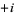and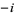can then be distinguished. Since either choice is possible, there is no ambiguity in definingas "the" square root of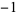." https://mathworld.wolfram.com/PrincipalSquareRoot.html

"The principal square root of a numberis denoted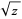(as in the positive real case) and is returned by the Wolfram Language function Sqrt[z]." https://mathworld.wolfram.com/SquareRoot.html

Last edited:
•PeroK
fresh_42 said:
As usual, I like the version on nLab:
https://ncatlab.org/nlab/show/square+root
I don't think I do for this seems to imply that ## x = \sqrt 4 ## [edit: has a unique solution identifies a unique number] in ## \mathbb R ## but not in ## \mathbb Z ##, however we have rehearsed our differences on foundations of mathematics before and I don't think it would be productive to do so againLast edited: